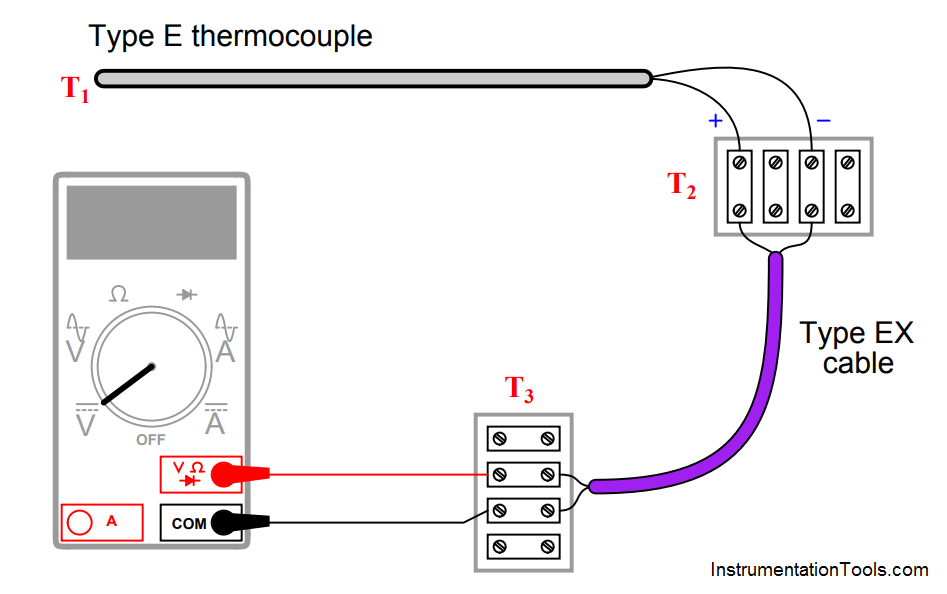# Type E thermocouple Calculations

Type E thermocouple

Calculate the amount of voltage “seen” by the voltmeter given the following measurement and reference junction temperatures:• T1 = 233 oC ; T2 = 31 oC ; T3 = 25 oC ; Vmeter = __________ mV
• T1 = 348 oC ; T2 = 40 oC ; T3 = 16 oC ; Vmeter = __________ mV
• T1 = −161 oC ; T2 = −4 oC ; T3 = 23 oC ; Vmeter = __________ mV
• T1 = 836 oC ; T2 = 34 oC ; T3 = 19 oC ; Vmeter = __________ mV

All answers based on ITS-90 thermocouple table values:

• T1 = 233 oC ; T2 = 31 oC ; T3 = 25 oC ; Vmeter = 14.395 mV
• T1 = 348 oC ; T2 = 40 oC ; T3 = 16 oC ; Vmeter = 23.856 mV
•  T1 = −161 oC ; T2 = −4′oC ; T3 = 23 oC ; Vmeter = −9.039 mV
• T1 = 836 oC ; T2 = 34 oC ; T3 = 19 oC ; Vmeter = 62.701 mV

Note: all temperatures at T2 are irrelevant because this is a junction between similar metals (type E wires connecting to corresponding type EX extension wires). The reference (cold) junction is where the type EX wires connect to copper, at temperature T3.